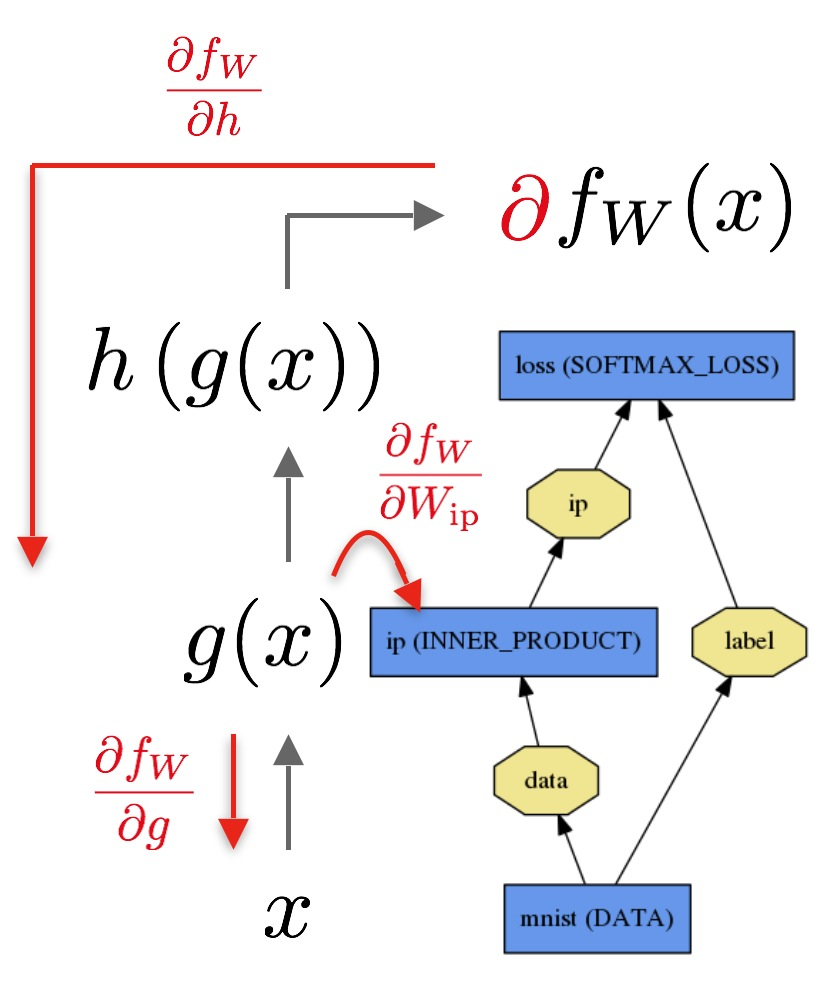# 如何用C++实现自己的Tensorflow

## 为什么？## Tensorflow

TensorFlow是由Google开源的一个深度学习库。在TensorFlow的内核，有一个大的组件，将操作串在一起，行成一个叫做 运算符图 的东西。这个运算符图是一个有向图G=(V,E)$G = (V,E)$,在某些节点u1,u2,,un,vV$u_1,u_2,…,u_n,v \in V$e1,e2,,enE,ei=(ui,v)$e_1,e_2,…,e_n \in E, e_i = (u_i,v)$ 存在某些运算符将u1,,un$u_1,…,u_n$ 映射到 v$v$

## 超级简单的概述

f(x,y) = x * y

df(x,y)dx=y$\frac{df(x,y)}{dx} = y$

df(x,y)dy=x$\frac{df(x,y)}{dy} = x$

f(x1,x2,...,xn)=f(x)=xTx$f(x_1,x_2,...,x_n) = f(\mathbf{x}) = \mathbf{x}^T\mathbf{x}$

df(x)dxi=2xi$\frac{df(\mathbf{x})}{dx_i} = 2x_i$

xf(x)=2x$\nabla_x f(\mathbf{x}) = 2\mathbf{x}$

df(g(h(x)))dx=df(g(h(x)))dg(h(x))dg(h(x))dh(x)dh(x)x$\frac{df(g(h(x)))}{dx} = \frac{df(g(h(x)))}{dg(h(x))} \frac{dg(h(x))}{dh(x)} \frac{dh(x)}{x}$

## 5分钟内反向模式

x -> h -> g -> f

dx <- dh <- dg <- df## 实施

class var {
// Forward declaration
struct impl;

public:
// For initialization of new vars by ptr
var(std::shared_ptr<impl>);

var(double);
var(const MatrixXd&);
var(op_type, const std::vector<var>&);
...

// Access/Modify the current node value
MatrixXd getValue() const;
void setValue(const MatrixXd&);
op_type getOp() const;
void setOp(op_type);

// Access internals (no modify)
std::vector<var>& getChildren() const;
std::vector<var> getParents() const;
...
private:
// PImpl idiom requires forward declaration of the     class:
std::shared_ptr<impl> pimpl;
};

struct var::impl{
public:
impl(const MatrixXd&);
impl(op_type, const std::vector<var>&);
MatrixXd val;
op_type op;
std::vector<var> children;
std::vector<std::weak_ptr<impl>> parents;
};


enum class op_type {
plus,
minus,
multiply,
divide,
exponent,
log,
polynomial,
dot,
...
none // no operators. leaf.
};


class expression {
public:
expression(var);
...
// Recursively evaluates the tree.
double propagate();
...
// Computes the derivative for the entire graph.
// Performs a top-down evaluation of the tree.
void backpropagate(std::unordered_map<var, double>& leaves);
...
private:
var root;
};


backpropagate(node, dprev):
derivative = differentiate(node)*dprev
for child in node.children:
backpropagate(child, derivative)


## Eigen

Eigen的代码看起来像：

Matrix A(...), B(...);
auto lazy_multiply = A.dot(B);
typeid(lazy_multiply).name(); // the class name is something like Dot_Matrix_Matrix.
Matrix(lazy_multiply); // functional-style casting forces evaluation of this matrix.


Eigen库是非常强大的，这就是为什么它是tensorflow自我使用的主要后台。这意味着除了这种惰性计算技术之外，还有其他方面的优化。

## 运算符重载

// These 3 lines code up an entire neural network!
var sigm1 = 1 / (1 + exp(-1 * dot(X, w1)));
var sigm2 = 1 / (1 + exp(-1 * dot(sigm1, w2)));
var loss = sum(-1 * (y * log(sigm2) + (1-y) * log(1-sigm2)));


## 基准

1. Tensorflow的神经网络 23812.5 ms
2. Scikit的神经网络库： 22412.2 ms
3. Autodiff的神经网络，迭代，优化： 25397.2 ms
4. Autodiff的神经网络，具有迭代，无优化： 29052.4 ms
5. Autodiff的神经网络，具有递归，无优化： 28121.5 ms©️2019 CSDN 皮肤主题: 大白 设计师: CSDN官方博客